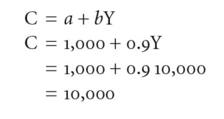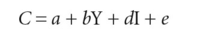# regression analysis

(redirected from Ordinary least squares regression)
Also found in: Dictionary, Thesaurus, Medical, Acronyms, Encyclopedia.

## Regression analysis

A statistical technique that can be used to estimate relationships between variables.

## Regression Analysis

In statistics, the analysis of variables that are dependent on other variables. Regression analysis often uses regression equations, which show the value of a dependent variable as a function of an independent variable. For example, a regression could take the form:

y = a + bx

where y is the dependent variable and x is the independent variable. In this case, the slope is equal to b and a is the intercept. When plotted on a graph, y is determined by the value of x. Regression equations are charted as a line and are important in calculating economic data and stock prices.

## regression analysis

The measurement of change in one variable that is the result of changes in other variables. Regression analysis is used frequently in an attempt to identify the variables that affect a certain stock's price.

## regression analysis

a statistical technique for estimating the equation which best fits sets of observations of dependent variables and independent variables, so generating the best estimate of the true underlying relationship between these variables. From this estimated equation it is then possible to forecast what the (unknown) dependent variable will be for a given value of the (known) independent variable.

Regression analysis is used in SALES FORECASTING to estimate trend lines in time series analysis and causal links with variables which affect sales; and in ECONOMETRICS to measure relationships between economic variables.

Collins Dictionary of Business, 3rd ed. © 2002, 2005 C Pass, B Lowes, A Pendleton, L Chadwick, D O’Reilly and M Afferson

## regression analysis

a statistical technique used in ECONOMETRICS for estimating the EQUATION that best fits sets of observations of DEPENDENT VARIABLES and INDEPENDENT

VARIABLES, so generating the best estimate of the true underlying relationship between these variables. From this estimated equation, it is then possible to redict what the (unknown) dependent variable(s) will be for a given value of the (known) independent variable(s).

Taking the simplest example of a linear equation with just one independent variable and a dependent variable (disposable income and consumption expenditure), the problem is to fit a straight line to a set of data consisting of pairs of observations of income (Y) and consumption. Fig. 168 shows such a set of paired observations plotted in graph form, and we need to find the equation of the line that provides the best possible fit to our data, for this line will yield the best predictions of the dependent variable. The line of best fit to the data should be chosen so that the sum of the squares of the vertical deviations (distances) between the points and the line should be a minimum. This method of ordinary least squares is applied in most regressions. The goodness of fit of the regression line to the sample observations is measured by the CORRELATION COEFFICIENT.

In arithmetic terms the line depicted in Fig. 168 is a linear equation of the form:where the coefficients of the equation, a and b, are estimates (based on single observations) of the true population parameters. These constants, a and b, obtained with the method of ordinary least squares, are called the estimated regression coefficients, and once their numerical values have been determined then they can be used to predict values of the dependent variable from values of the independent variable (Y). For example, if the estimated regression coefficient of a and b were 1,000 and 0.9 respectively then the regression equation would be C=1,000 + 0.9 Y and we could predict that for a disposable income of £10,000, consumer expenditure would be:The regression coefficient of the slope of the linear regression, b, is particularly important in economics for it shows the change in the dependent variable (here consumption) associated with a unit change in the independent variable (here income). For example, in this case a b value of 0.9 suggests that consumers will spend 90% of any extra disposable income.

The regression equation will not provide an exact prediction of the dependent variable for any given value of the independent variable, because the regression coefficients estimated from sample observations are merely the best estimate of the true population parameters and are subject to chance variations.

To acknowledge the imperfections in any estimated regression equation based on a sample in depicting the true underlying relationship in the population as a whole, the regression equation is generally written as:with the addition of a residual or error term, e , to reflect the residual effect of chance variations and the effects of other independent variables (such as, say, interest rates on consumer credit) that influence consumption spending but are not explicitly included in the regression equation.

Where it is felt that more than one independent variable has a significant effect upon the dependent variable, then the technique of multiple linear regression will be employed. The technique involves formulating a multiple linear regression equation involving two or more independent variables, such as:where I is the interest rate on consumer credit and d is an additional regression coefficient attached to this extra independent variable. Estimation of this multiple linear regression equation by the method of ordinary least squares involves fitting a three-dimensional plane or surface to a set of sample observations of consumer spending, disposable income and interest rates in such a way as to minimize the squared deviations of the observations from the plane.

In arithmetic terms, the sample observations can be used to generate numerical estimates of the three regression coefficients (a , b and d) in the above equation. See FORECASTING.

Collins Dictionary of Economics, 4th ed. © C. Pass, B. Lowes, L. Davies 2005
References in periodicals archive ?
Composite policy: ordinary least squares regression on decision outcomes scale for all participants Variable [Beta] Criticality of subordinates' tasks and skills .65(*) (positive value favours non-critical subordinate) Supervisory status (positive value favours non-supervisor) .20(*) Subordinate sex (positive value favours female subordinate) .05 Unpaid leave -.66(*) Vary site .56(*) Child care -.27(*) Elder care .20(*) (F7,42) 73.33(*) [R.sup.2] .92 [[R.sup.2].sub.adj] .91 * p [less than] .001.
Table 2 contains the results of ordinary least squares regression analyses for the long-term care and ambulatory populations.
Ordinary least squares regression is easy in APL, because * performs regression directly between a lead and trailing argument as follows: C*Y 1.00
Data analysis included frequencies, means, one sample t-tests, ANOVA, and ordinary least squares regression. Findings include the fact that while tutors did view the use of computers to be motivating for their tutees, they did not believe that learning was enhanced.
What can one say about cross-country foreign direct investment (FDI) using panel data and ordinary least squares regressions? This book has it all.
Findings in ordinary least squares regressions indicated that occupational portrayals of males are most likely to appear in magazines with a high male audience.
Dynamic ordinary least squares regressions are a statistically efficient method for estimating the long-run response of relative export prices to each of these variables (Box B).
Appended are: (1) State indicators for demographic and financial variables; (2) The impact of state financial strategies on high school graduation rates; and (3) Ordinary least squares regressions.
Table : TABLE III Weighted Ordinary Least Squares Regressions Explaining the Net Private Saving Rate (S/Y) With and Without a Measure of the Perceived Likelihood of World War Within Ten Years

Site: Follow: Share:
Open / Close